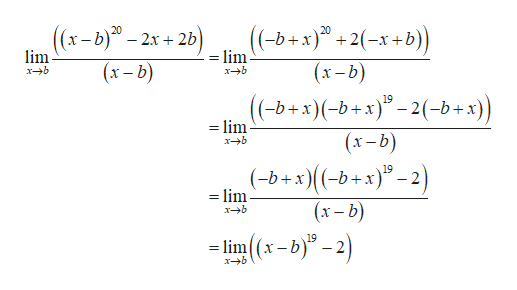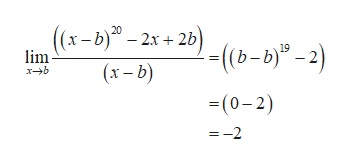Find the following limit or state that it does not exist. Assume b is a fixed real number.((x-b)^(20)-2x+2b)/(x-b)

Question

Find the following limit or state that it does not exist. Assume b is a fixed real number.

((x-b)^(20)-2x+2b)/(x-b)

Step 1

Obtain the limit assuming b is a fixed number as,help_outlineImage Transcriptionclose(-b+x) +2-x+b) 20 ((x-b)-2x2b) lim =lim (x-b) (x-b) x-b xb (-b+x)(-b+x)-2(-b+x) =lim 19 (x-b) xb (-b+x((-b+x)2 (x-b) 19 - 2 = lim x-b =lim fullscreen
Step 2

On further simpli...help_outlineImage Transcriptionclose((x-b)-2x+2)(b-b)-2) 19 lim (x-b) xb (0-2) =-2 fullscreen

Want to see the full answer?

See Solution

Want to see this answer and more?

Our solutions are written by experts, many with advanced degrees, and available 24/7

See Solution
Tagged in

Other